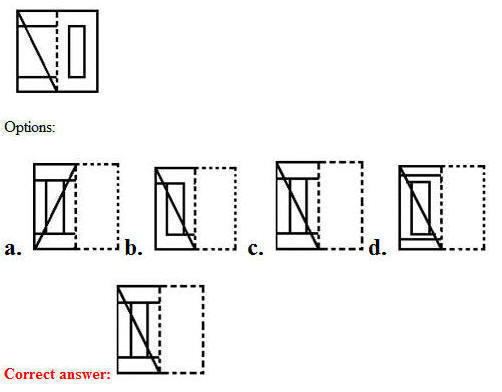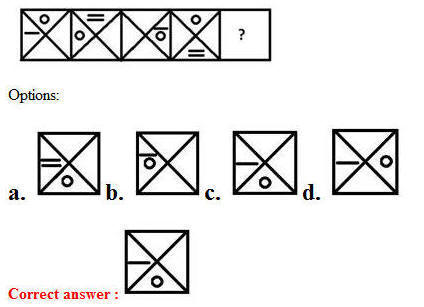# 05 July 2019 – Morning Shift (Reasoning)

SSC CHSL (Tier – 1) Online Exam Paper – 2018 “held on 05 July 2019”

Morning Shift (Reasoning)

Q1. Three persons A, B and C have different amounts of rupees with them. If A takes ₹6 from C, A will have equal amount as B has. A and B together have total ₹74. How many rupees does B have?

Options:

1. 44
2. 40
3. 34
4. 30

Q2. Select the set in which the numbers are related in the same way as are the numbers of the following set.
(5, 7, 11)

Options:

1. (23, 29, 31)
2. (15, 17, 23)
3. (17, 19, 21)
4. (14, 16, 18)

Correct answer : (23, 29, 31)

Q3. Select the combination of letters that when sequentially placed in the gaps of the given letter series will complete the series.
s_ _m r q c t_p d m _ q _ t s _ _ m r q c t

Options:

1. rdsrcrd
2. pdsrcrd
3. pdsrbpd
4. pdsrcpd

Q4. Two statements are given, followed by three conclusions numbered I, II and III. Assuming the statements to be true, even if they seem to be at variance with commonly known facts, decide which of the conclusions logically follow(s) from the statements.

Statements:
All purses are rupees.
Some rupees are coins.

Conclusions:
I. Some purses are coins.
II. No purse is coin.
III. No rupee is coin.

Options:

1. Either Conclusion I or II follows.
2. Only Conclusion II and III follow.
3. Only Conclusion I and II follow.
4. Only Conclusion I and III follow.

Correct answer : Either Conclusion I or II follows.

Q5. Which number will replace the question mark (?) in the following series?
16, 18, 21, 26, 33, ?

Options:

1. 45
2. 43
3. 46
4. 44

Q6. Select the set in which the numbers are related in the same way as are the numbers of the following set.
(2, 4, 8)

Options:

1. (4, 16, 64)
2. (1, 2, 6)
3. (5, 25, 625)
4. (3, 9, 34)

Correct answer : (4, 16, 64)

Q7. Select the number-pair in which the two numbers are related in the same way as are the two numbers of the following number-pair.
19 : 380

Options:

1. 14 : 196
2. 13 : 182
3. 12 : 146
4. 17 : 289

Correct answer : 13 : 182

Q8. Select the Venn diagram that best illustrates the relationship between the following classes.
Fuel, Diesel, LiquidQ9. Three of the following four words are alike in a certain way and one is different. Pick the odd word out.

Options:

1. History
2. Political Science
3. Sociology
4. Biology

Q10. A figure of transparent sheet with a pattern is given below. Select the option that shows how the pattern would appear when the transparent sheet is folded at the dotted line.Q11. ‘Kidney’ is related to ‘Excretion’ in the same way as ‘Heart’ is related to ‘_________’.

Options:

1. Blood Circulation
2. Heart Chambers
3. Human body
4. Artery

Q12. How many triangle are present in the given figure?Options:

1. 23
2. 25
3. 22
4. 24

Q13. Select the correct mirror image is the given figure when the mirror is placed to the right of the figure.Q14. Two different positions of the same dice are shown below, the six faces of which are numbered 1 to 6. find the number on the face opposite to the one having ‘5’Options:

1. 1
2. 4
3. 3
4. 2

Q15. Select the word-pair in which the two words are related in the same way as are the two words in the following word-pair.
Zinc : Brass

Options:

1. Copper : Bronze
2. Gold : Ornament
3. Mercury : Thermometer
4. Silver : Ring

Correct answer : Copper : Bronze

Q16. A+ B means: ‘A is husband of B’
A– B means: ‘A is brother of B’
A×B means: ‘A is sister of B’
A÷B means: ‘A is mother of B’.

If P + R ÷ S × M – K, then how is R related to K?

Options:

1. Mother
2. Daughter
3. Paternal grandmother
4. Sister

Q17. In a code language, VERBATIM is written as EUHYPLWD. How will SALESMAN be written as in that language?

Options:

1. OHVDDQVP
2. HODVQDPV
3. VDOHQDPV
4. HODVVPDQ

Q18. Three of the following four numbers are alike in a certain way and one is different. Pick the number that is different from the rest.

Options:

1. 50
2. 195
3. 170
4. 65

Q19. Select the option that is related to the third letter-cluster in the same way as the second letter-cluster is related to the first letter-cluster.
MDUK : QHYO : : SALQ : ?

Options:

1. WEPV
2. WEPU
3. VDPV
4. WDPU

Q20. Three of the following four letter-clusters are alike in a certain way and one is different. Pick the odd one out.

Options:

1. HLPT
2. CGKQ
3. DHLP
4. XBFJ

Q21. Which two signs should be interchanged in the following equation to make it correct?
405 + 27 × 40 – 308 ÷ 22 = 314

Options:

1. + and ÷
2. ÷ and ×
3. + and –
4. × and +

Correct answer : + and ÷

Q22. If YOGHURT is coded as 7678392, then how will QUICKLY be coded as?

Options:

1. 8393237
2. 7383237
3. 8393127
4. 8393117

Q23. Select the figure that will come next in the following figure series.Q24. Arrange the following activities in a logical and meaningful order.

1. Return journey
2. Travel arrangements
3. Hotel stay
4. Sightseeing
5. Reaching destination

Options:

1. 3, 2, 5, 4, 1
2. 2, 5, 3, 1, 4
3. 2, 5, 3, 4, 1
4. 5, 3, 4, 2, 1

Correct answer : 2, 5, 3, 4, 1

Q25. Select the option in which Figure-X is embedded. (Rotation is not allowed)